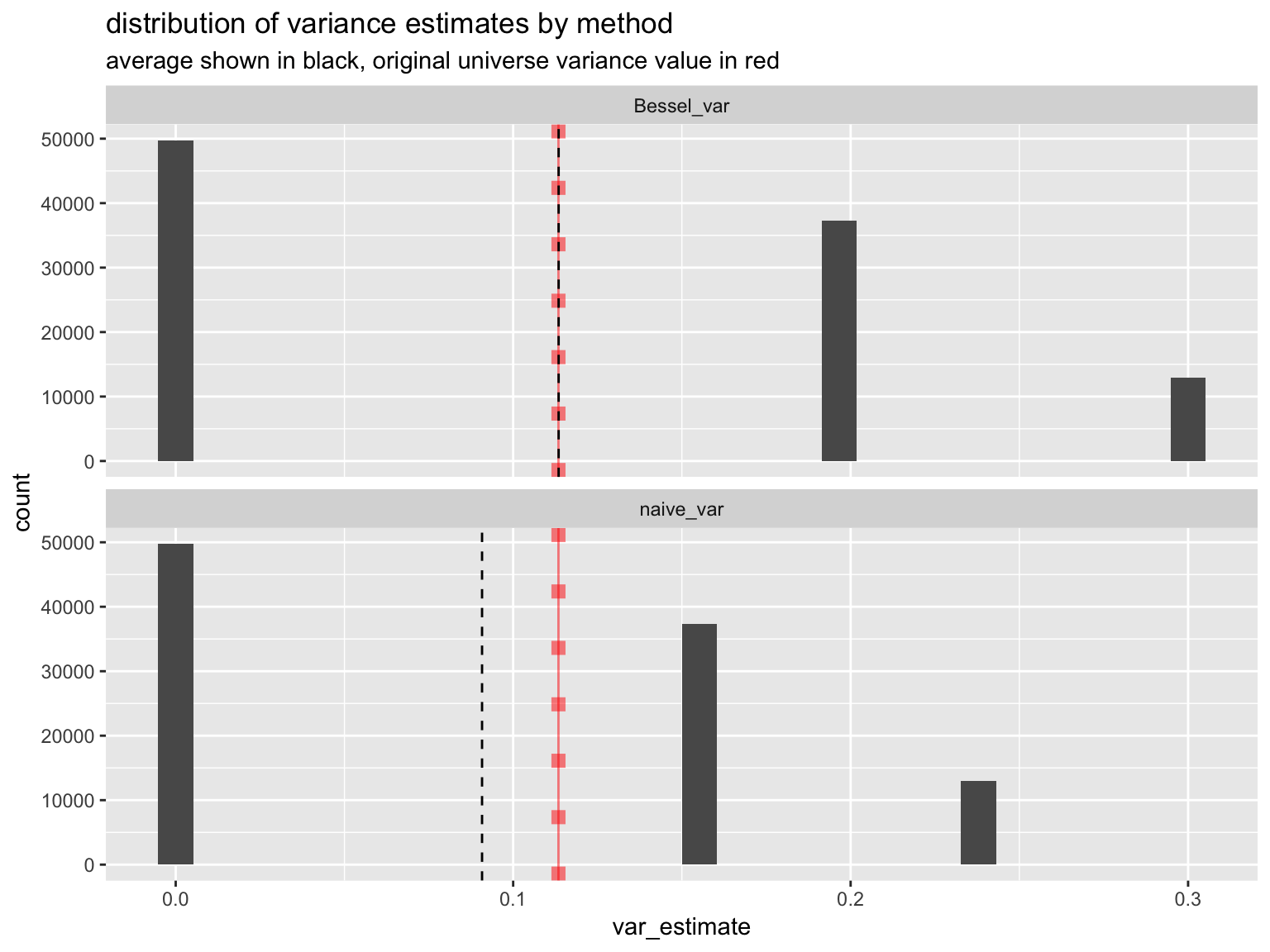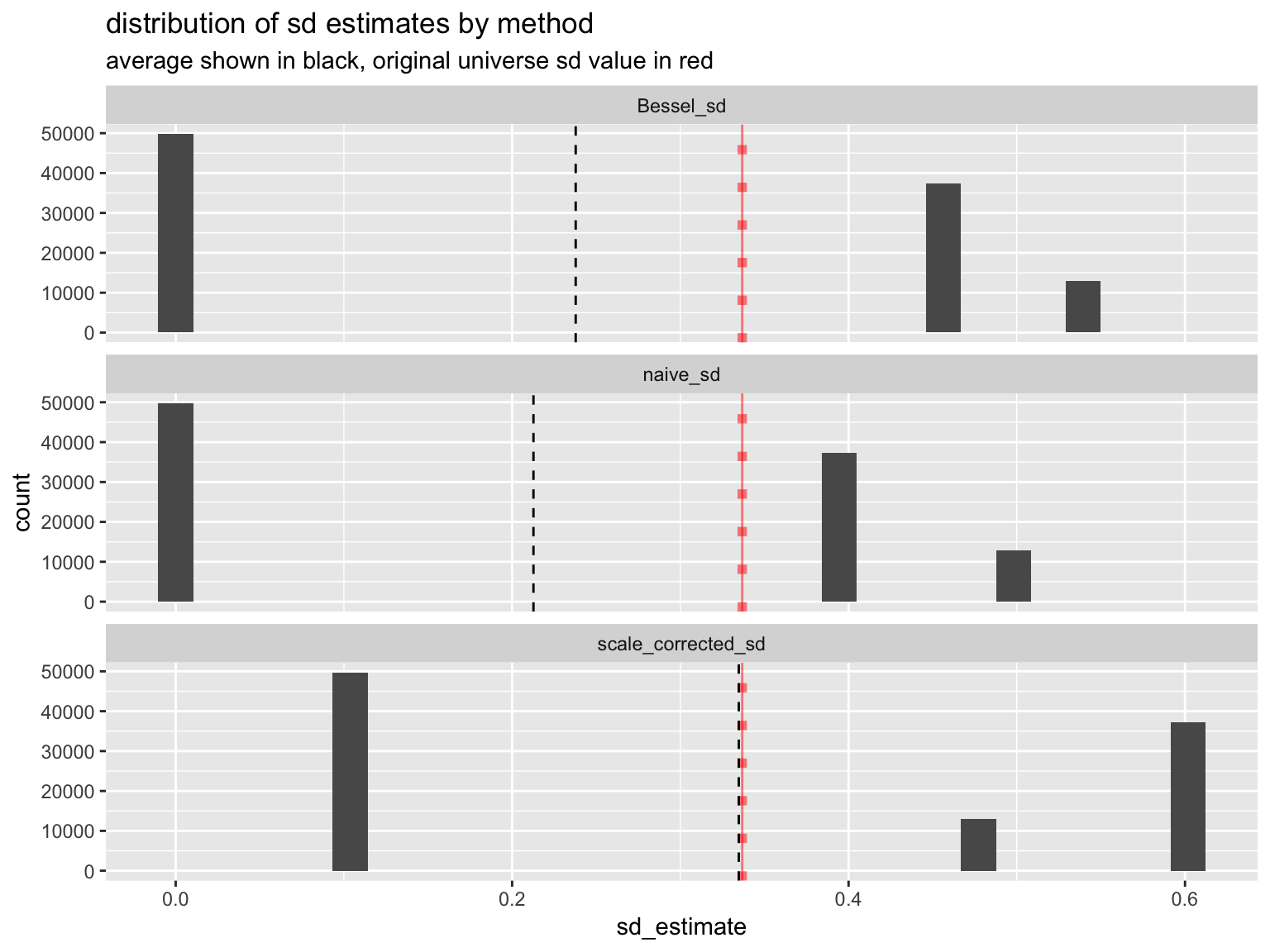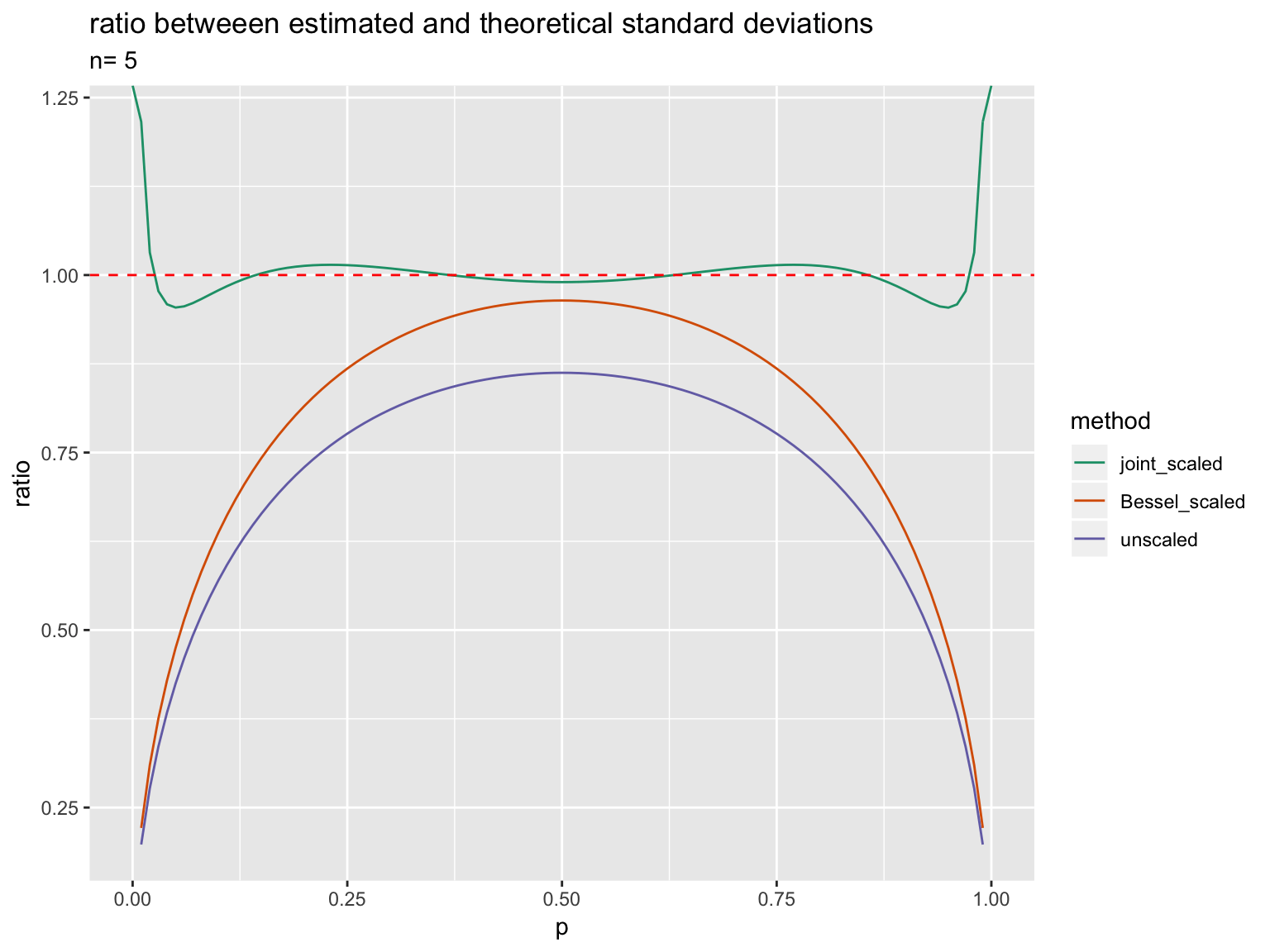# How to de-Bias Standard Deviation Estimates

This note is about attempting to remove the bias brought in by using sample standard deviation estimates to estimate an unknown true standard deviation of a population. We establish there is a bias, concentrate on why it is not important to remove it for reasonable sized samples, and (despite that) give a very complete bias management solution.

Everitt’s The Cambridge Dictionary of Statistics 2nd Edition, Cambridge University Press defines a “statistic” as:

A numeric characteristic of a sample.

Informally we can say a statistic is a summary. And this lets us say the field of statistics is the science of relating the observed summaries of samples to the corresponding unobserved summary of the total population or universe the samples are drawn from.

For example: we can take our universe to be the set of female adult crew members of the Titanic, and our observable to be if they survived or not. Then our universe or total population is the following:

```  20 survivors  (we will code these as 1's)
3 fatalities (we will code these as 0's)
```

We can ask R to show us some common summaries: mean (which we will denote as “p”), variance, and standard_deviation.

```universe = c(rep(1, 20), rep(0, 3))

p = mean(universe)
print(p)
#  0.8695652

variance <- mean((universe - p)^2)
print(variance)
#  0.1134216

standard_deviation <- sqrt(variance)
print(standard_deviation)
#  0.3367812
```

Note, we deliberately did not call R’s `var()` and `sd()` methods, as they both include Bessel’s sample correction. For an entire universe (sometimes called a population) the sample correction is inappropriate, and leads to a wrong answer.

Python’s statistics library has a sensible approach. It presents un-corrected (population or universe versions) and corrected (sample versions) on equal footing:

The bias in question is falling off at a rate of `1/n` (where `n` is our sample size). So the bias issue loses what little gravity it ever may have ever had when working with big data. Most sources of noise will be falling off at a slower rate of `1/sqrt(n)`, so it is unlikely this bias is going to be the worst feature of your sample.

But let’s pretend the sample size correction indeed is an important point for a while.

Under the “no bias allowed” rubric: if it is so vitally important to bias-correct the variance estimate, would it not be equally critical to correct the standard deviation estimate?

The practical answer seems to be: no. The straightforward standard deviation estimate itself is biased (it has to be, as a consequence of Jensen’s inequality). And pretty much nobody cares, corrects it, or teaches how to correct it, as it just isn’t worth the trouble.

Let’s convert that to a worked example. We are going to investigate the statistics of drawing samples of size 5 (uniformly with replacement) from our above universe or population. To see what is going on we will draw a great number of examples (though in practice when we could do this, we could more easily directly summarize over this small universe).

Here is the distribution of observed sample variances for both the naive calculation (assuming the variance observed on the sample is representative of the universe it was draw from) and the Bessel corrected calculation (inflating the sample estimate of variance by `n/(n-1)`, or 25% in this case). The idea is: the observable Bessel corrected variances of samples converge to the unobserved un-corrected (or naive) variance of the population.We generated the above graph by uniformly drawing samples (with replacement) of size 5 from the above universe. The experiment was repeated 100000 times and we are depicting the distribution of what variance estimates were seen as a density plot (only a few discrete values occur in small samples). The black dashed vertical line is the mean of the 100000 variance estimates, and the vertical red line and blocks structure indicates the true variance of the original universe or population.

Notice the Bessel corrected estimate of variance is itself a higher variance estimate: many of the adjusted observations are further away from the true value. The Bessel correction (in this case) didn’t make any one estimate better, it made the average over all possible estimates better. In this case it is inflating all the positive variance estimates to trade-off against the zero variance estimates.

Now suppose we run the same experiment for standard deviation estimates.The Bessel adjustment helped, but was nowhere near correcting the bias. Both estimates of standard deviation are severely downward biased.

Here is a that experiment again, including an additional correction procedure (which we will call joint scale corrected).Notice the scale corrected estimate is unbiased. We admit, if this were so massively important it would be taught more commonly. However, as standard deviations summaries are more common than variance summaries (example: `summary.lm()`): having an unbiased estimate for a standard deviation is probably more important than having an unbiased estimate for variance.

The scale corrected estimate did raise the variance of the estimation procedure, but not much more than the Bessel correction did:

```     estimation_method variance_of_estimate
1: scale_corrected_sd           0.05763954
2:           naive_sd           0.04554781
3:          Bessel_sd           0.05693477
```

How did we correct the standard deviation estimate?

It is quite simple:

1. For a binomial outcome and sample size `n`, the standard deviation estimate is essentially a lookup table that maps the pair `n` (the sample size) and `k` (the number of 1’s seen) to an estimate. Call this table `est(n,.)`. Remember `est(n,.)` is just a table of `n+1` numbers.
2. The expected value of our estimator is just going to be the sum of the probability of each observable situation times the estimate we make in that situation. This is supposed to match the unknown true standard deviation which is `sqrt(p(1-p))` (assuming we exactly knew `p`). This means for any `p` we want these two quantities near each other, or:`dbinom(k, n, p)` being the probability of drawing “`k`” 1’s from in n draws when 1’s have a probability of p (this is a known quantity, a value we just copy in). More examples of using this methodology can be found here.
3. We ask an optimizer to pick values for `est(n,.)` that simultaneously make many of these check equations near true. We used a discrete set of `p`‘s with a Jeffreys prior style example weighing. One could probably recast this as a calculus of variations problem over all `p`s and work closer to an analytic solution.

The concept is from signal processing: observation is an averaging filter, so we need to design an approximate inverse filter (something like an unsharp mask). The above inverse filter was specialized for the 0/1 binomial case, but one can use the above principles do design inverse filters for additional situations.

All of the above was easy in R and we got the following estimation table (here shown graphically with the un-adjusted and also Bessel adjusted estimation procedures).

In the above graph: `n` is the sample size, `k` is how many 1’s are observed, and the y axis is the estimate of the unknown true population standard deviation.Notice the “joint scaled” solution wiggles around a bit.

As we have said, the above graph is the estimator. For samples of size 5 pick the estimator you want to use and the k corresponding to how many 1’s you saw in your sample: then the y height is the estimate you should use for population standard deviation.

In the next graph: `p` is the unknown true frequency of 1’s, and the `y`-axis is the difference between the expected value of the estimated standard deviation and the true standard deviation.Notice the joint scaled estimate reports a non-zero standard deviation even for all zeros or all ones samples. Also notice the joint estimate tries to stay near the desired difference of zero using a smooth curve that wiggles above and below the desired difference of zero.

The next graph is the same sort of presentation for ratios of estimated to true standard deviations.We still see the Bessel corrected estimates are better than naive, but not as good as jointly adjusted.

And that is how you correct standard deviation estimates (at least for binomial experiments). We emphasize that nobody makes this correction in practice, and for big data and large samples the correction is entirely pointless. The variance correction is equally pointless, but since it is easier to perform it is usually added.

Categories: Opinion Pragmatic Data Science Tutorials### jmount

Data Scientist and trainer at Win Vector LLC. One of the authors of Practical Data Science with R.